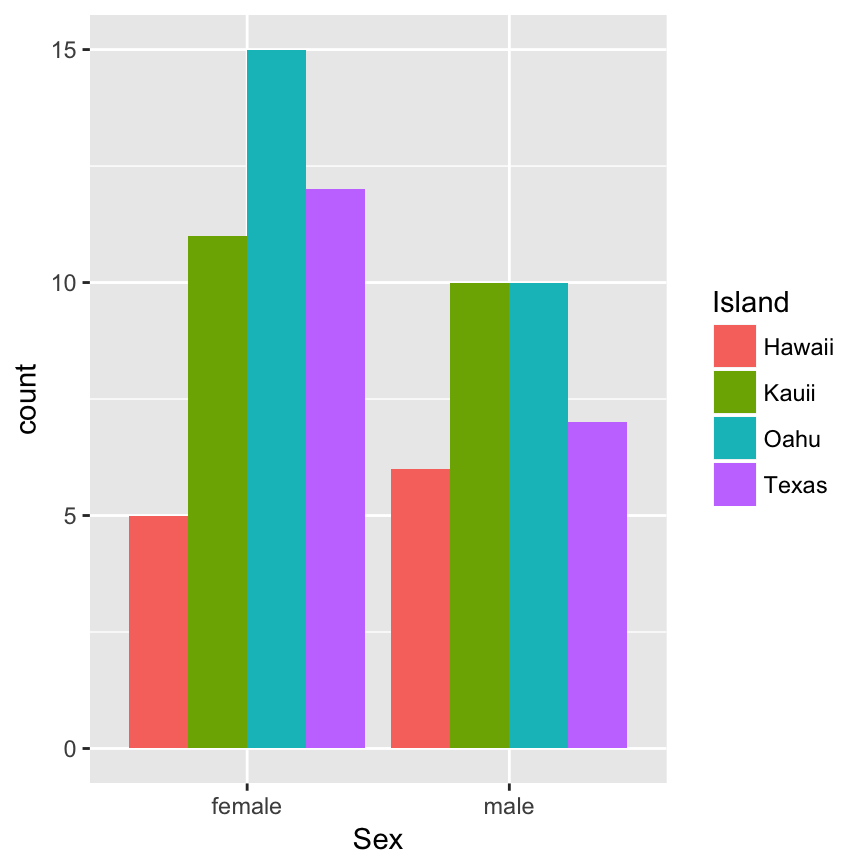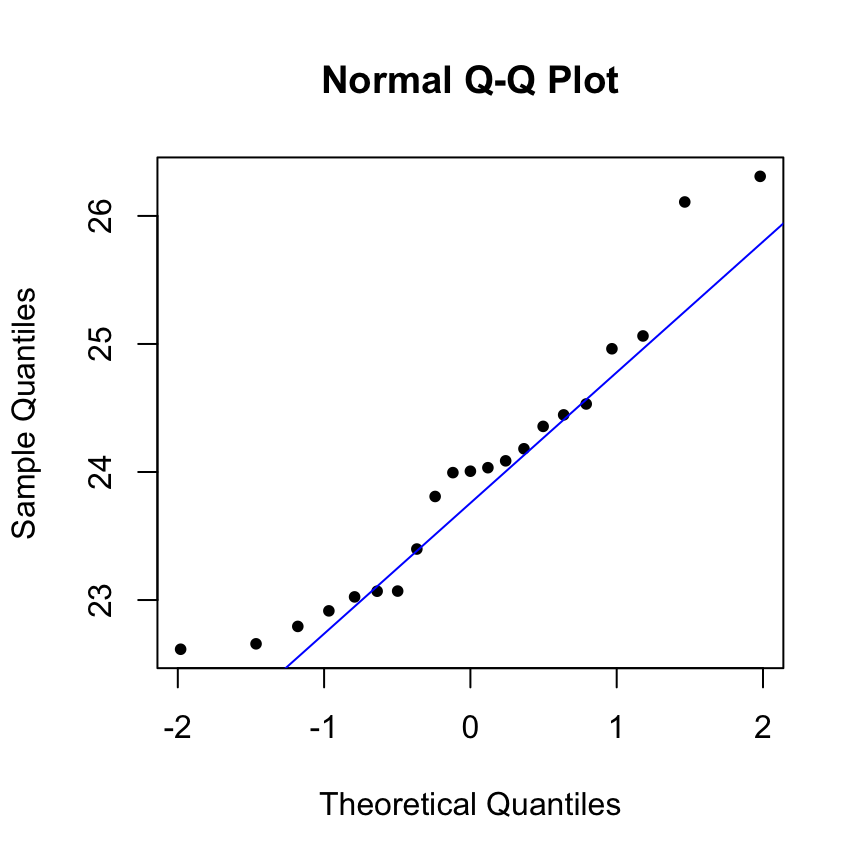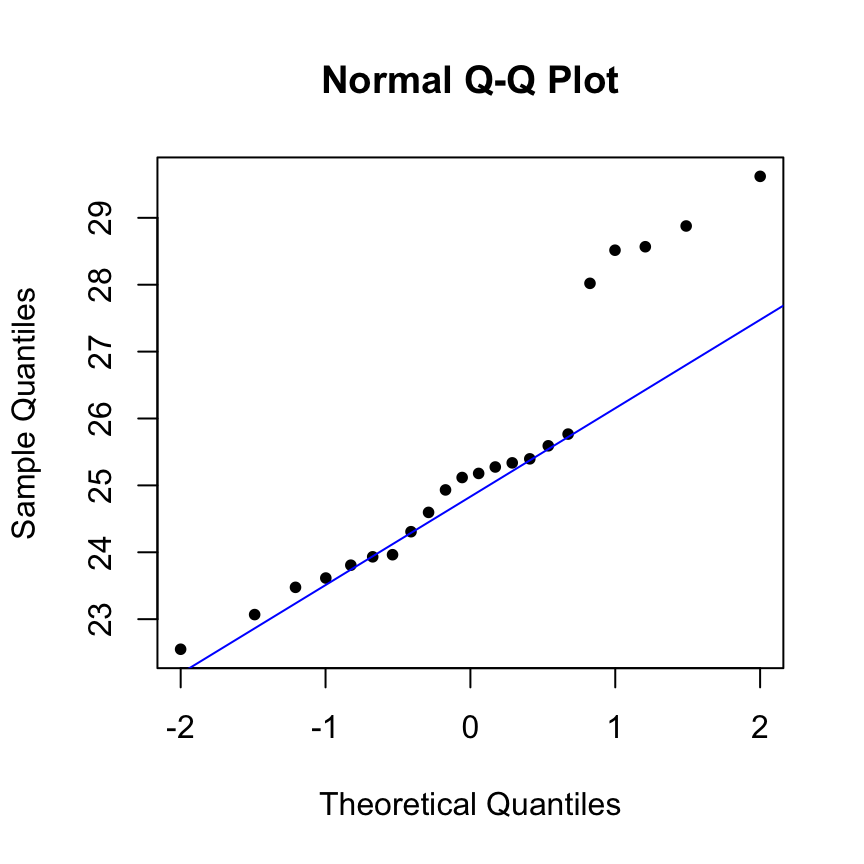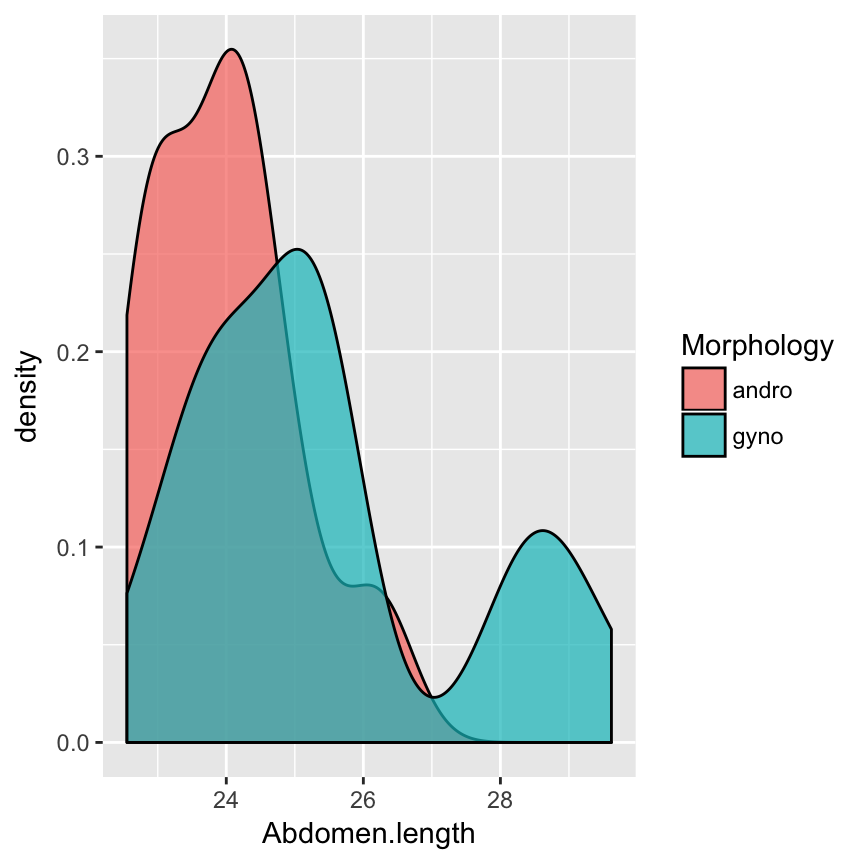## Final Project EXAMPLE

### BIO5312

Josiah S. Carberry

This document shows an example project, using the Kauii damselfly dataset (last known whereabouts, Probability homework). Note that while this example is shown only with three questions, your project must have FOUR QUESTIONS.

## Introduction

For this project, I analyze the dataset of results from a study of color pattern differences in the damselfly Ischnura ramburii. These damselflies display unique pigmentation: all males are blue-green, while some females are orange and some are blue-green like the males. The orange females are referred to as “gynomorphs” (female-like morphs) and the blue-green females are referred to as “andromorphs” (male-like morphs). This data contains information about these damselflies collected from the Hawaiian islands Oahu, Kauai, and Hawaii, as well as from Texas.

These variables are contained in the dataset:

• Island, (Categorical) The collection location of the damselfly specimen.
• Sex, (Categorical) The sex of the damselfly specimen
• Morphology, (Categorical) The morphology of the damselfly specimen
• Wing.size, (Numeric), The wing size, in unit pixels, of the damselfly specimen
• Mating.status, (Categorical), The mating status of the damselfly specimen
• Abdomen.length, (Numeric), The abdomen length, in unit mm, of the damselfly specimen

## Data Preparation

No preparation was needed as the dataset was already tidy.

damsel <- read.csv("damselfly.csv")

## Questions

#### Question 1: Do males and females occur in equal proportions across collection sites?

To address this question, I will use a contingency-table analysis:

## Determine contingency table values
damsel %>%
group_by(Sex, Island) %>%
tally() 
## # A tibble: 8 x 3
## # Groups:   Sex [?]
##      Sex Island     n
##   <fctr> <fctr> <int>
## 1 female Hawaii     5
## 2 female  Kauii    11
## 3 female   Oahu    15
## 4 female  Texas    12
## 5   male Hawaii     6
## 6   male  Kauii    10
## 7   male   Oahu    10
## 8   male  Texas     7
damsel.table <- rbind(c(5,11,15,12), c(6, 10,10, 7))

## Confirm that expected counts are sufficiently high to meet assumptions (they are)
chisq.test(damsel.table)$expected  ## [,1] [,2] [,3] [,4] ## [1,] 6.223684 11.881579 14.14474 10.75 ## [2,] 4.776316 9.118421 10.85526 8.25 ## Now run and examine test output chisq.test(damsel.table) ## ## Pearson's Chi-squared test ## ## data: damsel.table ## X-squared = 1.1586, df = 3, p-value = 0.763 My null hypothesis is that sex and island are unassociated. My alternative hypothesis is that sex and island are associated. I confirmed assumptions were met for a chi-squared contingency table analysis by checking expected counts: Only 1 expected count in the table was less than 5, and all were greater than 1. The chi-squared test gave a test-statistic of 1.1586 and P=0.763, which is higher than $$\alpha$$. Therefore I fail to reject the null hypothesis, and I have no evidence that sex and island are associated. In conclusion, my analysis suggested that sex are evenly distributed across collection sites. To accompany this analysis, I made a barplot of damselfly counts across sex and island. My plot shows that all counts are below 15. It appears that there are more males from Hawaii compared to females, but for all other Islands, there were relatively more females collected than males. ## Visualize ggplot(damsel, aes(x = Sex, fill = Island)) + geom_bar(position = "dodge")#### Question 2: Among females, do gynomorphs and andromorphs tend to have the same abdomen lengths? To address this question, I will use a Wilcoxon rank sum test ### Determine sample size: There are 21 andro and 22 gyno. This is small, we should check assumptions to see if t-test is usable: damsel %>% filter(Sex == "female") %>% group_by(Morphology) %>% tally() ## # A tibble: 2 x 2 ## Morphology n ## <fctr> <int> ## 1 andro 21 ## 2 gyno 22 ### Check normal assumption, since small N damsel %>% filter(Morphology == "andro") -> andro damsel %>% filter(Morphology == "gyno") -> gyno qqnorm(andro$Abdomen.length, pch=20)
qqline(andro$Abdomen.length, col = "blue")qqnorm(gyno$Abdomen.length, pch=20)
qqline(gyno$Abdomen.length, col = "blue")### Run wilcoxon text wilcox.test(andro$Abdomen.length, gyno$Abdomen.length) ## ## Wilcoxon rank sum test ## ## data: andro$Abdomen.length and gyno\$Abdomen.length
## W = 129, p-value = 0.01258
## alternative hypothesis: true location shift is not equal to 0
## determine directionality with medians
damsel %>%
filter(Sex == "female") %>%
group_by(Morphology) %>%
summarize(median(Abdomen.length))
## # A tibble: 2 x 2
##   Morphology median(Abdomen.length)
##       <fctr>                    <dbl>
## 1      andro                 24.00616
## 2       gyno                 25.14836

My null hypothesis is that andro and gyno morphs tend to have the same abdomen lengthse. My alternative hypothesis is that andro and gyno morphs tend to have different abdomen lengths. There were 21 andromorphs and 22 gynomorphs, which are relatively small sample size. I used normal QQ plots to check their distributions. The andromorph plot was mostly normal, but the gynomorph plot showed strong deviations at higher values. Small sample size coupled with poor normality means a non-parametric test should be used. The Mann-Whitney U test gave a test-statistic of 129 and P=0.01258, which is lower than $$\alpha$$. Therefore I reject the null hypothesis, and I have evidence that gyno and andromorphs have different abdomen lengths. Looking at the actual median abdomen lengths, I find that andromorphs are generally shorter (24 mm) than gynomorphs (25.1 mm). In conclusion, my analysis suggested gynomorphs have significantly longer, albeit only by about 1 mm, abdomens compared to andromorphs.

To accompany this analysis, I made a density plot of andromorph and gynomorph abdomen lengths. My plot shows that andromorphs have generally lower lengths with a single peak around 24, and that gynomorphs have a bimodal distribution with peaks around 25 and 28.

## Visualize
damsel %>%
filter(Sex == "female") %>%
ggplot(aes(x = Abdomen.length, fill = Morphology)) + geom_density(alpha=0.7)#### Question 2: Can variables in this dataset be used to predict wing size?

To address this question, I will use a linear model.

## use step() function to determine "optimal" predictors for a model, with AIC criterion.
model <- step(lm(Wing.size ~ ., data=damsel), trace=0)
tidy(model)
##             term   estimate std.error statistic      p.value
## 1    (Intercept)  403.72318 81.705988   4.94117 4.884965e-06
## 2 Morphologygyno   43.09491 16.219509   2.65698 9.704817e-03
## 3 Morphologymale -195.81840 14.300524 -13.69309 9.887484e-22
## 4 Abdomen.length   72.80252  3.376914  21.55889 3.919822e-33
glance(model)
##   r.squared adj.r.squared    sigma statistic      p.value df    logLik
## 1 0.9281418     0.9251477 50.65094  309.9911 4.492627e-41  4 -404.0816
##        AIC      BIC deviance df.residual
## 1 818.1631 829.8168 184717.3          72

I constructed a linear model to predict wing size, using the step() function to ascertain optimal predictors and find the model with the best AIC. The final model contained two predictors: abdomen length and morphology, all of which were highly significant. The model has a significant intercept of 403.72 (P=4.88e-6), meaning that an andromorph damselful with 0 abdomen length will have a wing size of 403.72 mm. The gynomorph coefficient is 43.09 (P=9.7e-3), which means that a gynomorph will have on average 43.09 mm longer wing size compared to andromorphs. The male coefficient is -195.8 (P=9.89e-22), which means that a male will have on average 195.8 mm shorter wing size compared to andromorphs. The abdomen length coefficient is 72.8 (P=3.91e-33), so the predictors explain roughly 92% of the variation in wing size. Therefore, this is a very strong and robust model and this dataset can strongly predict wing size.

To accompany this analysis, I made a scatterplot of wing size against abdomen length, with points colored by morphology and regression lines through each morphology.My plot shows a clear linear relationship between wing size and abdomen length, and there does not appear to be an interaction between abdomen and morphology. In general, females have larger wings than males. The figure suggests that gynomorph females may have slightly larger wing sizes compared to andromorph females.

## visualize model
ggplot(damsel, aes(x = Abdomen.length, y  = Wing.size, color = Morphology)) + geom_point() + geom_smooth(method = "lm")